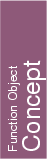## Kernel::ConstructPlane_3

A model for this must provide:

Kernel::Plane_3
 fo.operator() ( Kernel::RT a, Kernel::RT b, Kernel::RT c, Kernel::RT d)
creates a plane defined by the equation a px + b py + c pz + d = 0. Notice that is degenerate if a = b = c.

Kernel::Plane_3
 fo.operator() ( Kernel::Point_3 p, Kernel::Point_3 q, Kernel::Point_3 r)
creates a plane passing through the points p, q and r. The plane is oriented such that p, q and r are oriented in a positive sense (that is counterclockwise) when seen from the positive side of the plane. Notice that is degenerate if the points are collinear.

Kernel::Plane_3
 fo.operator() ( Kernel::Point_3 p, Kernel::Direction_3 d)
introduces a plane that passes through point p and that has as an orthogonal direction equal to d.

Kernel::Plane_3
 fo.operator() ( Kernel::Point_3 p, Kernel::Vector_3 v)
introduces a plane that passes through point p and that is orthogonal to v.

Kernel::Plane_3 fo.operator() ( Kernel::Line_3 l, Kernel::Point_3 p)
introduces a plane that is defined through the three points l.point(0), l.point(1) and p.

Kernel::Plane_3 fo.operator() ( Kernel::Ray_3 r, Kernel::Point_3 p)
introduces a plane that is defined through the three points r.point(0), r.point(1) and p.

Kernel::Plane_3
 fo.operator() ( Kernel::Segment_3 s, Kernel::Point_3 p)
introduces a plane that is defined through the three points s.source(), s.target() and p.

### Refines

AdaptableFunctor (with two arguments)